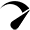# The solution to CS50 psets 2 substitution problem (2022)## CS50 psets 2 substitution problem (2022)

For the cs50 psets 2 substitution problem we have to write a program that implements substitution cipher. The output should be as shown below.

``````\$ ./substitution JTREKYAVOGDXPSNCUIZLFBMWHQ
plaintext:  HELLO
ciphertext: VKXXN``````

## Substitution Cipher

In a substitution cipher, we “encrypt” a message by replacing every letter in the message with another letter. To do that, we use a key. So, in this case, mapping each of the letters of the alphabet to the letter it should correspond to when we encrypt it.

In order to decrypt the message, the receiver of the message would need to know the key. Using that key they can reverse the process and translate the encrypted text (ciphertext) back into the original message (plaintext).

#### Example:

A key might be a string like this NQXPOMAFTRHLZGECYJIUWSKDVB. This 26-character key means that A (the first character of the alphabet) is converted into N (the first character of the key). Z ( the last letter of the alphabet) should be converted into B ( the last character of the key). Like that, each and every letter in the alphabet is converted into the corresponding character of the key.

## Code

So, here is my solution to this problem.

``````#include <cs50.h>
#include <stdio.h>
#include <string.h>
#include <ctype.h>

void substitution(string key);
void substitution_value(char position, string key);

int main(int argc, string argv[])
{
if (argc == 2 ) // checks if there is only 2 strings
{
if (strlen(argv) == 26) // checks if the argument contain only 26 characters
{
for (int i = 0; i < strlen(argv); i++) // loop through the argument string
{
if (!isalpha(argv[i])) // checks if there is only alphabetical characters in the key string
{
printf("Key must contain 26 characters.\n");
return 1;
}

// checks if the key contains repeated characters
for (int j = i + 1; j < strlen(argv); j++)
{
if(toupper(argv[i]) == toupper(argv[j]))
{
printf("Key must not contain repeated characters.\n");
return 1;
}
}
}

// execute the substitution function
substitution(argv);

}
else
{
printf("Key must contain 26 characters.\n");
return 1;
}
}
else
{
printf("Usage: ./substitution key\n");
return 1;
}

}

// substitution function
void substitution(string key)
{
//get plaintext and store it in plaintext string
string plaintext = get_string("plaintext: ");

// get the length of the plaintext and store it in an integer
int plaintext_length = strlen(plaintext);

printf("ciphertext: ");
// iterate through the plaintext string
for (int k = 0; k < plaintext_length; k++)
{
// checks if the plantext has only alphabatical characters
if (isalpha(plaintext[k]))
{
char plaintext_character = plaintext[k];
if(islower(plaintext_character))
{
substitution_value(tolower(plaintext_character), key);
}
else
{
substitution_value(toupper(plaintext_character), key);
}
}
else
{
printf("%c", plaintext[k]);
}
}
printf("\n");
}

void substitution_value(char position, string key)
{
string abc = "ABCDEFGHIJKLMNOPQRSTUVWXYZ";

for (int i = 0; i < strlen(abc); i++)
{
if (islower(position))
{
if (position == tolower(abc[i]))
{
printf("%c", tolower(key[i]));
}
}
else
{
if (position == toupper(abc[i]))
{
printf("%c", toupper(key[i]));
}
}
}
}``````

You may also like to read: The solution to cs50 labs 2 scrabble problem (2022)

(Visited 380 times, 1 visits today)

### One ResponseOptimized by Seraphinite Accelerator